## Flux (Surface Integrals of Vectors Fields)

Derivation of formula for Flux

Suppose the velocity of a fluid in xyz space is described by the vector field F(x,y,z). Let S be a surface in xyz space. The flux across S is the volume of fluid crossing S per unit time. The figure below shows a surface S and the vector field F at various points on the surface.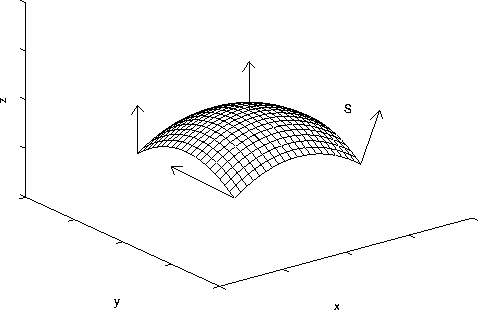What is the formula for the flux? Let us consider the flux through an infinitesimal piece of the surface with area dS above the point (x_0,y_0) in the xy plane. (The surface is denoted by the dotted region.) Let n denote the unit normal vector to the surface. Let us suppose that the velocity vector F(x_0,y_0,z_0) of the fluid makes an angle theta with the unit normal. In one unit of time a blob of fluid of length |F|=|F(x_0,y_0,z_0)| will pass through the surface. (The blob is in the shape of a parallelepiped.)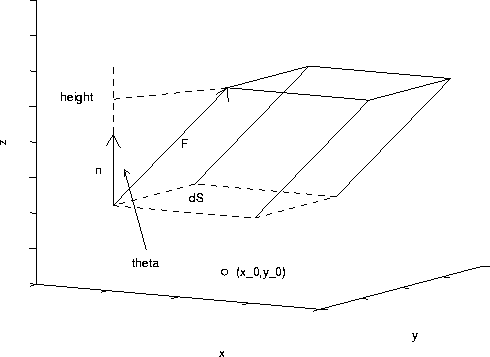The volume of this fluid is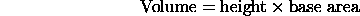The base area is dS. The height is equal to |F|cos(theta). Hence, we have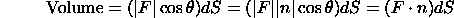Here we have used the fact that |n|=1 and the property of the dot product. The total flux through the surface is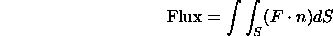This is a surface integral.

We can write the above integral as an iterated double integral. Suppose that the surface S is described by the function z=g(x,y), where (x,y) lies in a region R of the xy plane. The unit normal vector on the surface above (x_0,y_0) (pointing in the positive z direction) is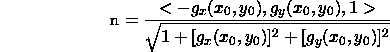The surface area of an infinitesimal piece of the surface above a infinitesimal region in the xy plane with area dA containing (x_0,y_0) is given by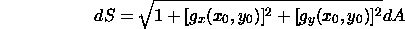Hence, it follows that the total flux is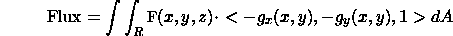If we are asked for the flux in the negative z direction, then we use the vector <g_x(x,y),g_y(x,y),-1> for the normal direction.

Formula for Flux for Parametric Surfaces

Suppose that the surface S is described in parametric form: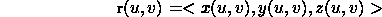where (u,v) lies in some region R of the uv plane. It can be shown that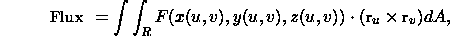Here, x means the cross product. Note, one may have to multiply the normal vector r_u x r_v by -1 to get the correct direction.

Example

Find the flux of the vector field <y,x,z> in the negative z direction through the part of the surface z=g(x,y)=16-x^2-y^2 that lies above the xy plane (see the figure below).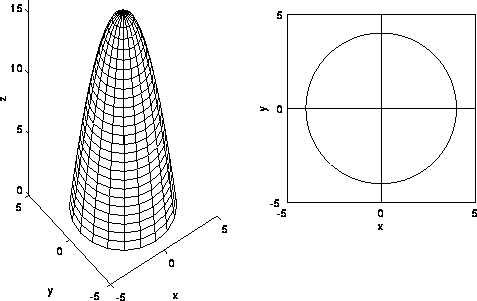For this problem: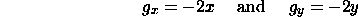It follows that the normal vector is <-2x,-2y,-1>. Computing Fo<-2x,-2y,-1>, we have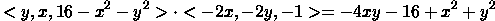Here we use the fact that z=16-x^2-y^2. Hence, the integral becomes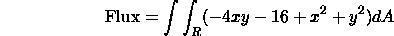The region R is the disk of radius 4 centered at the origin shown below. It is convenient to convert to polar coordinates. The region of integration is 0<=r<=4 and 0<=theta<=2*pi. Substituting,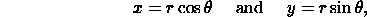we have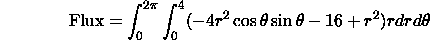Here we have used the fact that x^2+y^2=r^2. (We can also integrate with respect to theta first, then r.) The inner integral with respect to r yields (remember theta is held constant)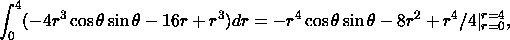which is equal to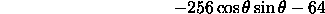The inner integral is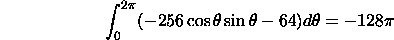Hence, the flux through the surface in the downward z direction is -128*pi cubic units per unit time. This means a negative amount of fluid crosses the surface in the downward direction. In other words, there is a flux of 128*pi cubic units per unit time in the upward z direction.

[Vector Calculus Home] [Math 254 Home] [Math 255 Home] [Notation] [References]